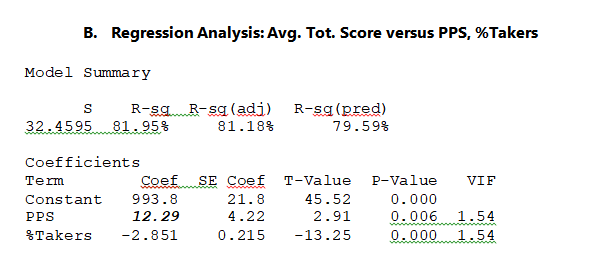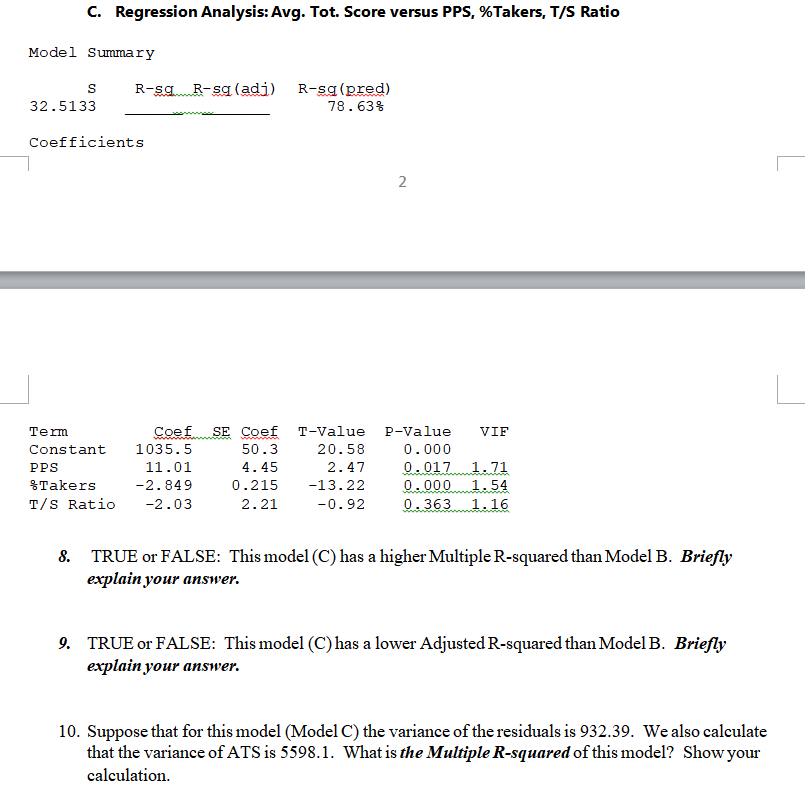# C. Regression Analysis: Avg. Tot. Score versus PPS, %Takers, T/S Ratio Model Summary SR-sg R-sq (...

Model B is below.C. Regression Analysis: Avg. Tot. Score versus PPS, %Takers, T/S Ratio Model Summary SR-sg R-sq (adi) R-sq (pred) 78. 63% 32.5133 Coefficients Term Constant 1035.5 PPS %Takers T/S Ratio -2.03 Coef T-Value P-Value VIF 50.3 4.45 20.58 0.000 11.01 -2.849 0.215 13.22g.0001 54 2.21 -0.92 TRUE or FALSE: This model (C) has a higher Multiple R-squared than Model B. Briefly explainyour answer 8. 9. TRUE or FALSE: This model (C) has a lower Adjusted R-squared than Model B. Briefly explain your answer 10. Suppose that for this model (Model C) the variance of the residuals is 932.39. We also calculate Showyour that the variance of ATS is 5598.1. What is the Multiple R-squared of this model? calculation

(8) True, model C has a higher Multiple R-squared value than Model B, because in linear regression, every time we add a new variable to the model, the Multiple R-squared value of the model always keeps on increasing.

(9) True, mode C has a lower Adjusted R-squared value than Model B, because in linear regression, the Adjusted R-squared value decreases if we add an insignificant variable to the model. Here, the variable "T/S ratio" is insignificant to model C. Hence, the Adjusted R-squared value has decreased.

(10) Multiple R-squared value for Model C = 1 - (932.39/5598.1) = 0.8334 = 83.34%